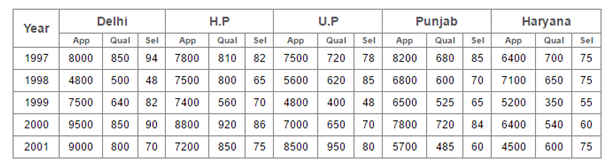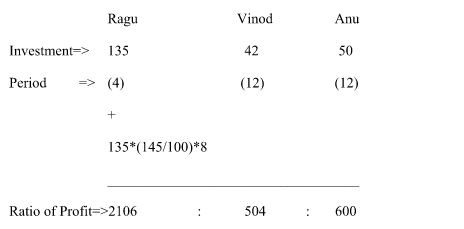# “20-20” Quantitative Aptitude | Crack SBI Clerk 2018 Day-33

Dear Friends, SBI Clerk 2018 Notification has been released we hope you all have started your preparation. Here we have started New Series of Practice Materials specially for SBI Clerk 2018. Aspirants those who are preparing for the exams can use this “20-20” Quantitative Aptitude Questions.

## Daily Practice Test Schedule | Good Luck

 Topic Daily Publishing Time Daily News Papers & Editorials 8.00 AM Current Affairs Quiz 9.00 AM Logical Reasoning 10.00 AM Quantitative Aptitude “20-20” 11.00 AM Vocabulary (Based on The Hindu) 12.00 PM Static GK Quiz 1.00 PM English Language “20-20” 2.00 PM Banking Awareness Quiz 3.00 PM Reasoning Puzzles & Seating 4.00 PM Daily Current Affairs Updates 5.00 PM Data Interpretation / Application Sums (Topic Wise) 6.00 PM Reasoning Ability “20-20” 7.00 PM English Language (New Pattern Questions) 8.00 PM General / Financial Awareness Quiz 9.00 PM

[WpProQuiz 1384]

Click “Start Quiz” to attend these Questions and view Explanation

### Click Here for SBI Clerk Prelims and Mains 2018 – Full Length Mock Test

1. (3.82)3 × (16.13)2 ÷ √ (4095.89) = 4x
1. 6
2. 7
3. 4
4. 3
5. 5
1. 18.23 × 21.04 ÷? +352 = 429.07
1. 5
2. 18
3. 7
4. 12
5. None of these
1. 154 % of 524.999 + 12.85 % of 1389.73 =?
1. 1100
2. 990
3. 850
4. 1050
5. None of these
1. ?2 + 44.78% of 1695.99 +359 = 5320.729
1. 55
2. 57
3. 61
4. 65
5. None of these
1. (8/15) of 5027.98 + (11/200) of 58344.5 =?
1. 6784
2. 7856
3. 5890
4. 4786
5. None of these

Direction (Q. 6 – 10): What will come in the place of question mark (?) in the following number series?

1. 4, 6, 10, 14, ? , 26, 34
1. 18
2. 22
3. 23
4. 16
5. None of these
1. 4, 9, 35, 167, 899,?
1. 3459
2. 4367
3. 4785
4. 5519
5. None of these
1. 28, 30, 64, 198, 800, 4010, ?
1. 8976
2. 15690
3. 13684
4. 21562
5. None of these

1. 56, 84, ? , 189, 283.5, 425.25
1. 96
2. 108
3. 126
4. 156
5. None of these
1. 28, 16, 10, 7, ? , 4.75
1. 5.5
2. 3.5
3. 4
4. 4.25
5. None of these
1. The sum of money 2 times itself in 5 years at simple interest. The same amount and same rate of interest, 8 times will be in?
1. 32 years
2. 35 years
3. 26 years
4. 28 years
5. None of these
1. A box contains 4 red balls, 3 white balls, 6 black balls. If two balls are drawn out random, then the probability that both balls are either red or black balls
1. 7/26
2. 17/26
3. 3/26
4. 11/26
5. None of these
1. The length and the breadth of rectangular plot are in the ratio of 7:5. If the cost of fencing the plot @ 25 per meter is Rs.7200, then find the area of the plot?
1. 6240 Sq meter
2. 3560 Sq meter
3. 4560 Sq meter
4. 5040 Sq meter
5. None of these
1. Ragu, Vinod and Anu enter into a Partnership with shares in the ratio of (9/2) : (7/5) : (5/3). After four months Ragu increases his share by 45%. If the total profit at the end of the year be Rs. 44940, what will be the share of Vinod in the profit?
1. Rs.7216
2. Rs.7448
3. Rs.6780
4. Rs.8956
5. Rs.7056
1. P, Q and R can fill the tank in 15, 18 and 20 min respectively. All three began to fill the tank together but P and Q left 4 and 5 min respectively before filling the tank. In how much time does R takes to fill the tank?
1. 8  30/31 min
2. 7  26/33 min
3. 9  30/33 min
4. 6  26/31 min
5. None of these

Directions (Q. 16 – 20): Study the following table and answer the questions based on it.

Number of Candidates Appeared, Qualified and Selected in a Competitive Examination from Five States Delhi, H.P, U.P, Punjab and Haryana Over the Years 1997 to 2001.1. For which state the average number of candidates selected over the year is the maximum?
1. Delhi
4. Punjab
5. Haryana

1. In the year 1997, which state has the lowest percentage of candidates selected over the candidates appeared?
1. Delhi
3. Punjab
5. Haryana
1. The number of candidates selected from Haryana in all the years is approximately what percent of the number of candidates selected from Delhi in all the years?
1. 79.5%
2. 81%
3. 84.5%
4. 88.5%
5. 87.5%
1. What is the approximate percentage of total number of candidates selected to the total number of candidates qualified in all five states together during the year 1999?
1. 18%
2. 24%
3. 32%
4. 13%
5. 27%
1. What is the ratio between the total no of candidates appeared from all the states together during the year 1998 to 2001?
1. 248 : 349
2. 357 : 409
3. 318 : 349
4. 407: 529
5. None of these

Directions (Q. 1 – 5):

43 × 162 ÷ √ (4096) = 4x

(43 × 16 × 16)/64 = 4x

44 = 4x

X= 4

(18 × 21/x) + 352 = 429

18 × 21/x = 77

X= 18 × 21/77

X = 54/11= 5

154% of 525 + 13% of 1390 = x

(154 * 525)/100 + (13 * 1390)/100 = x

809 +182 = x

X= 991 ≈ 1000

X2 + (45 * 1696) /100 + 359 = 5321

X2 = 5321 -359 – 765

X2 = 4197

X ≈ 65

(8/15) of 5026 + (11/200) of 58344 = x

2680 + 3209 = x

X= 5889

Direction (Q. 6 – 10):

The pattern is, 2* Prime no’s (Starts with 2)

(ie., 2*2, 3*2, 5*2, 7*2, 11*2, 13*2, 17*2)

The pattern is, *2 + 13, *3 + 23, *4 +33, *5 + 43, *6 + 53

The pattern is, *1 + 2, *2 + 4, *3 + 6, *4 + 8, *5 + 10, *6 +12,..

The pattern is, *3/2

The pattern is, ÷ 2 × 2

The sum of money 2 times itself in 5 years. The same amount and the same rate of interest, the 8 times will be in,

Total amount= 2p

S.I = 2p-p =p

P*5*r/100 = p

R= 20%

P*20*n/100 = 7p

N= 35 years

Shortcut method:

=>(n-1)*t (here n is no of time, t is years)

=>(8-1)*5

=>35 years

P(E) = n(E)/n(S)

n(S) =  13C2

n(E) = 4C2 or 6C2

P(E) = 4C2 or 6C2 / 13C2

P(E) = (6+15)/78

P(E) = 7/26

The cost of fencing the plot @ 25 per meter is Rs. 7200

=>7200/25=Perimeter of Rectangle

=>288=2(7x+5x)

=>12x=144

=>x=12

The length and the breadth of rectangular plot are in the ratio of 8:6

Area of the plot= 84*60

=>5040 Sq meter.

Ragu, Vinod and Anu enter into a Partnership with shares in the ratio of (9/2) : (7/5) : (5/3)

=>(135:42:50)/30

=>135:42:50=>351 : 84 : 100

Vinod’s Share= (84/535)*44940 = 7056

(x-4)/ 15 + (x-5)/18 + x/20 =1

(12x-48+10x-50+9x)/180 =1

31x-98=180

31x= 278

X=278/31 = 8 30/31 min

Directions (Q. 16 – 20):

The average number of candidates selected over the given period for various states

For Delhi = 94 + 48 + 82 + 90 + 70/5 = 384/5 = 76.8

For HP = 82 + 65 + 70 + 86 + 75/5 = 378/5 = 75.6

For UP = 78 + 85 + 48 + 70 + 80/ = 361/5 = 72.2

For Punjab = 85 + 70 + 65 + 84 + 60/5 = 364/5 = 72.8

For Haryana = 75 + 75 + 55 + 60 + 75/5 = 340/5 = 68

Clearly the average is maximum for Delhi

The percentages of candidates selected over the candidates appeared in 1997, for various states are:

For Delhi = (94/8000 × 100)% = 1.175%

For HP = (82/7800 × 100)% = 1.051%

For UP = (78/7500 × 100)% = 1.040%

For Punjab = (85/8200 × 100)% = 1.037%

For Haryana (75/6400 × 100)% = 1.172%

Clearly, the percentage is lowest for Punjab

Required percentage = [(75 + 75 + 55 + 60 + 75) / (94 + 48 + 82 + 90 + 70) × 100]%

= [340/384 × 100]%

= 88.54% ≈ 88.5%

Required percentage = [(82 + 70 + 48 + 65 + 55)/(640 + 560 + 400 + 525 + 350)]%

= (320/2475 × 100)%

= 12.93% ≈ 13%

The total no of candidates appeared from all the states during the year 1998

= > 4800 + 7500 +5600 + 6800 +7100 = 31800

The total no of candidates appeared from all the states during the year 2001

= > 9000 + 7200 + 8500 + 5700 + 4500 = 34900

Respective ratio = 31800 : 34900 = 318 : 349